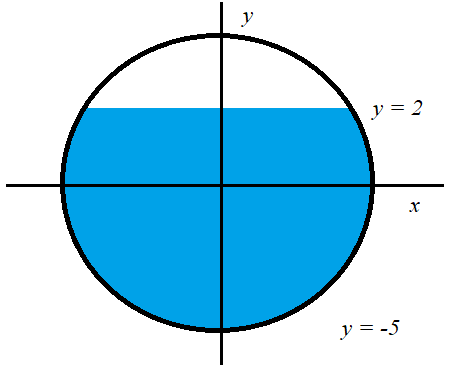# A water storage tank has the shape of a cylinder with diameter 10 ft. It is mounted so that the...

## Question:

A water storage tank has the shape of a cylinder with diameter {eq}10 \, \mathrm{ft} {/eq}. It is mounted so that the circular cross-sections are vertical. If the depth of the water is {eq}7 \, \mathrm{ft} {/eq}, find the percentage of the total capacity being used.

## Integration

Integration can be used to find area under the curve or to find the volume of solids. In solving the volumes of solid by integration, direct integration may not be the best approach and we need to do some integration techniques. In this specific problem we use technique called trigonometric substitution.

## Answer and Explanation:

The problem asks for the percentage of the total capacity used. We can solve for the full-capacity volume of the cylinder first.

{eq}V = \pi r^2 h \\ {/eq}

The radius is half the diameter which is 5 feet

{eq}V = \pi (5)^2 h \text{ ft} ^3 \\ V = 25 \pi h \text{ ft} ^3 \\ V = 78.5398 \text{ft}^3 {/eq}

We can draw the cylindrical tank such that its center is in the origin. This way we can define the circular base of the tank as

{eq}x^2+y^2 = r^2\\ x^2 + y^2= 5^2 {/eq}.

This is the equation of a circle with radius 5.

Shown below is the cross-section of the cylindrical tank. Since we placed the center of the tank at the origin, the bottom is located at y = -5 and the topmost filled part is at y = 2. This is because the height of the water is 7 feet. The task now is to integrate the equation of circle with the appropriate limits. And multiply the area with the length of the cylindrical tank to find the volumeThe figure is bounded by two curves {eq}x = \sqrt{ 5^2 - y^2} {/eq} to the right and {eq}x = -\sqrt{5^2 - y^2} {/eq} to the left. This means that to find the cross-sectional area we need to solve the integral

{eq}\displaystyle \text{ Area } = \int_{-5} ^ 2 \sqrt{ 5^2 - y^2} - (-\sqrt{5^2 - y^2}) dy \\ \displaystyle \text{ Area } =2 \int_{-5} ^ 2 \sqrt{ 5^2 - y^2} dy {/eq}

To solve the integral {eq}\displaystyle \int \sqrt{ 5^2 - y^2} dy \rightarrow \displaystyle 5 \int \sqrt{ 1 - \frac{y}{25}^2 }dy {/eq} we need to do trigonometric substitution.

We use the following substitution:

{eq}\displaystyle \frac{y}{5} = \sin \theta \\ \displaystyle \frac{1}{5} dy = \cos \theta d \theta {/eq}

{eq}\displaystyle 5 \int \sqrt{ 1 - \frac{y}{25}^2} dy \\ \displaystyle = 5 \int \sqrt{ 1 - \sin ^2 \theta } 5 \cos \theta d \theta \\ \displaystyle = 25 \int \sqrt{ 1 - \sin ^2 \theta} \cos \theta d \theta {/eq}

We use the identity {eq}\cos^2 \theta + \sin ^2 \theta = 1. {/eq}

{eq}\displaystyle 25 \int \sqrt{ 1 - \sin^ 2 \theta } \cos \theta d \theta \\ \displaystyle = 25 \int \sqrt{ \cos^2 \theta} \cos \theta d \theta \\ \displaystyle = 25 \int \cos \theta \cos \theta d \theta \\ \displaystyle = 25 \int \cos ^2 \theta d \theta {/eq}

Using the double angle identity for cosine.

{eq}\displaystyle \cos 2 \theta = \frac{1}{2} \bigg( 1 + \cos 2 \theta \bigg ) {/eq} to integrate the square of cosine.

{eq}\displaystyle 25 \int \cos ^2 \theta d \theta \\ \displaystyle = 25 \int \frac{1}{2} \bigg( 1 + \cos 2 \theta \bigg ) d \theta \\ \displaystyle = \frac{25}{2} \int \bigg( 1 + \cos 2 \theta \bigg ) d \theta \\ \displaystyle = \frac{25}{2}\bigg ( \theta + \frac{1}{2} \sin 2 \theta \bigg ) {/eq}

We can also use the double angle identity for sine to express the integral in simpler terms.

{eq}\sin 2 \theta = 2 \cos \theta \sin \theta {/eq}

{eq}\displaystyle \frac{25}{2}\bigg ( \theta + \frac{1}{2} \sin 2 \theta \bigg ) = \frac{25}{2}\bigg ( \theta + \cos \theta \sin \theta \bigg ) \\ {/eq}

The step is to rewrite the variables from {eq}\theta \rightarrow y {/eq}

{eq}\displaystyle \frac{25}{2}\bigg ( \theta + \cos \theta \sin \theta \bigg ) = \frac{25}{2}\bigg [ \sin ^{-1} \frac{y}{5} + \bigg ( \sqrt{ 1 -\frac{ y^2}{25}} \bigg ) \bigg( \frac{y}{5} \bigg) \bigg ] {/eq}

Therefore {eq}\displaystyle \int \sqrt{ 5^2 - y^2} dy = \frac{25}{2}\bigg [ \sin ^{-1} \frac{y}{5} + \bigg ( \sqrt{ 1 -\frac{ y^2}{25}} \bigg ) \bigg( \frac{y}{5} \bigg) \bigg ] {/eq}

Evaluating the with the limits.

{eq}\displaystyle \int_{-5}^2 \sqrt{ 5^2 - y^2} dy = \frac{25}{2}\bigg [ \sin ^{-1} \frac{y}{5} + \bigg ( \sqrt{ 1 -\frac{ y^2}{25}} \bigg ) \bigg( \frac{y}{5} \bigg) \bigg ] _{-5}^2 \\ \\ \displaystyle = \frac{25}{2}\bigg [ \sin ^{-1} \frac{2}{5} + \bigg ( \sqrt{ 1 -\frac{ 2^2}{25}} \bigg ) \bigg( \frac{2}{5} \bigg) \bigg ] - \frac{25}{2}\bigg [ \sin ^{-1} \frac{-5}{5} + \bigg ( \sqrt{ 1 -\frac{ (-5) ^2}{25}} \bigg ) \bigg( \frac{-5}{5} \bigg) \bigg ] \\ \displaystyle = \frac{25}{2}\bigg [ \sin ^{-1} \frac{2}{5} + \bigg ( \sqrt{ 1 -\frac{ 4}{25}} \bigg ) \bigg( \frac{2}{5} \bigg) \bigg ] - \frac{25}{2}\bigg [ \sin ^{-1} -1 + \bigg ( \sqrt{ 1 -\frac{25}{25}} \bigg ) \bigg( \frac{-5}{5} \bigg) \bigg ] \\ \displaystyle = \frac{25}{2}\bigg [ \sin ^{-1} \frac{2}{5} + \bigg ( \sqrt{ 1 -\frac{ 4}{25}} \bigg ) \bigg( \frac{2}{5} \bigg) \bigg ] - \frac{25}{2}\bigg [ \sin ^{-1} -1 \bigg ] \\ \displaystyle \int_{-5}^2 \sqrt{ 5^2 - y^2} dy = 29.361 \\ \displaystyle 2 \int_{-5} ^ 2 \sqrt{ 5^2 - y^2} dy = 58.723 {/eq}

This is the area of the cross-section filled with water. To find the volume, we multiply the area with length of the cylindrical tank, l.

{eq}V_{filled} = 58.723 l {/eq}

To find the percent of filled we divide the filled volume with the total volume.

{eq}\displaystyle \text{ percent filled } = \frac{58.723 l }{78.5398 l} \times 100 \% \\ \displaystyle \text{ percent filled } = 74.77 \% {/eq}